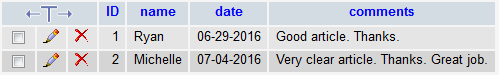﻿ How to Show the Structure of a MySQL Table Using PHP ﻿# How to Show the Structure of a MySQL Table Using PHPIn this article, we go over how to show the structure of a MySQL table using PHP.

By structure, we mean what columns the table is made up of and the data type and definition of those columns.

Thus, we can know the makeup, or structure, of the table.

The general format of the MySQL query to show the structure of a table is shown below.

So the following code above is the MySQL code to show the structure of the table named table_name.

We'll now show how to tie this in with PHP.

So, first, we must connect to the database.

After we do this, we create a variable named \$tablestructure. This \$tablestructure variable is set equal to a mysql_query() function that has the parameter "EXPLAIN table_name". This MySQL query is a query to show the structure of a table.

Since it's easier to visualize a table for these results, I put the data into table form.

So I create a table.

Right underneath this, I create a series of variables that stores data from the table. This is very important.

The name of each column of the table is in the first index of the array. So I create a variable named \$column_name and set it equal to \$data.

The data type and definition of each column of the table is in the second index of the array. So I created a variable named \$definition and set it equal to \$data.

The null value (whether the column is NULL or NOT NULL) is in the third index of the array. So I created a variable named \$null and set it equal to \$data.

The primary key value (whether the column is a primary key or not) is in the fourth index of the array. So I created a variable named \$primarykey and set it equal to \$data.

the default value (whether the column has a default value or not) is in the fifth index of the array. So I created a variable named \$default and set it equal to \$data.

The extra value (such as whether the column is set to auto_increment) is in the sixth index of the array. So I created a variable named \$extra and set it equal to \$data.

Cumulatively, all these variables represent the structure of a table. They represent the column name, its data type and definition, whether it is null or not, a primary key or not, has a default value or not, and whether it has an extra features such as auto_increment.

So now all of the table structure is in tabular form.

### Example

So below is a table called usercomments with the following table structure.Using the PHP code above, we can get the complete table structure of the above table. This is shown below.